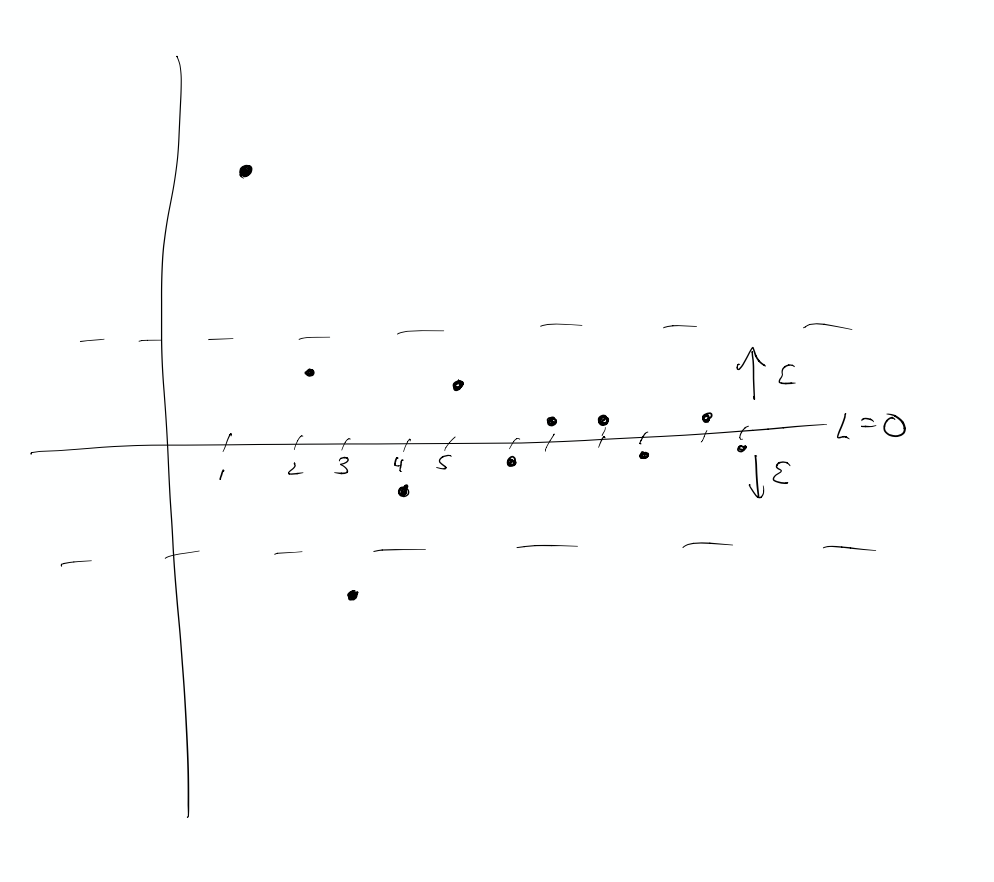# Sequences

Definition: An (infinite) sequence with rational coefficients is a function $$a:\mathbb{P}\to\mathbb{Q}$$. Normally we view it as the sequence $$a(1),a(2),\ldots$$.

Some examples:

• $$a(n)=0$$ for all $$n$$ (the zero sequence).
• $$a(n)=1/n$$ for $$n=1,2,\ldots$$
• $$a(n)=n$$ for $$n=1,2,\ldots$$
• $$a(n)=(-1)^n$$ for $$n=1,2,\ldots$$

We would like to have a way to speak about what happens to sequences as $$n$$ gets larger and larger (so for example the sequence grows, it bounces around, or it approaches a particular number.)

# Limit of a sequence

Definition: Let $$a(n)$$ be a sequence. Then we say that the limit of $$a(n)$$ is $$L$$ if, for every $$\epsilon>0$$, there is an integer $$N$$, so that $$|a(n)-L|<\epsilon$$ for all $$n\ge N$$. This is written:

$\lim_{n\to\infty} a(n)=L.$

and we say that the sequence converges to $$L$$.Examples

• Let $$a(n)$$ be the sequence defined by $$a(1)=1, a(2)=1/2, and a(n)=0$$ for $$n>2$$. Prove that the limit $$\lim_{n\to\infty} a(n)=0$$.
• Let $$a(n)$$ be the sequence $$a(n)=1/n$$. Prove that the limit of $$a(n)$$ as $$n\to\infty$$ is zero.
• Let $$a(n)=(-1)^{n}$$. Prove that the limit isn’t $$1$$. Then prove there is no limit.
• Let $$a(n)=n$$. Is there a limit?
• Let $$a(n)=(n+1)/n$$. Prove that the limit is $$1$$.
• Let $$a(n)=4+(-1/2)^{n}$$.

# Non-convergence

A sequence $$a(n)$$ does not converge to a limit $$L$$ means that - there exists $$\epsilon>0$$ such that - for all $$N$$ - there exists $$n\ge N$$ such that - $$|a(n)-L|>\epsilon$$

The sequence $$a(n)=(-1)^{n-1}$$ does not converge to any limit because no matter what $$L$$ you pick and what $$N$$ you choose the distance $$|(-1)^{n-1}-L|$$ bounces back and forth between $$|1-L|$$ and $$|1+L|$$ so if you choose $$\epsilon$$ smaller than the maximum of these two you satisfy the ‘non-convergence’ requirement.

# Limit rules make arguments standard

Proposition: If $$a(n)$$ converges to $$L$$ and $$b(n)$$ converges to $$M$$ then $$a(n)+b(n)$$ converges to $$L+M$$.

Proof: The estimation side calculation is that we can choose $$N$$ large enough that $$|a(n)-L|<\epsilon$$ and $$|b(n)-M|<\epsilon$$ for $$n\ge N$$. Then $$|a(n)+b(n)-L-M|<2\epsilon$$. So given $$\epsilon$$ we should choose $$N$$ large enought that $$|a(n)-L|<\epsilon/2$$ and similarly $$|b(n)-M|<\epsilon/2$$.

Proposition: Suppose that $$a(n)$$ is a sequence converging to $$L$$. Prove that there is an $$N$$ so that $$|a(n)|<2L$$ for $$n\ge N$$.

Proof: Choose $$\epsilon=L/2$$. Then there is an integer $$N$$ such that $$|a(n)-L|<L/2$$ for all $$n\ge N$$. This means that $$a(n)$$ is between $$L/2$$ and $$3L/2$$ so in particular it is less than $$2L$$.

Proposition: Suppose that $$a(n)$$ converges to $$L$$. Prove that $$a(n)^2$$ converges to $$L^2$$.

Proof: $$|a(n)^2-L^2|=|a(n)^2-a(n)L+a(n)L-L^2|\le |a(n)||a(n)-L| + |L||a(n)-L|$$. We can choose $$N$$ so that $$|a(n)|<2L$$ and $$N'$$ so that $$|a(n)-L|<\epsilon/4L$$. Then for $$n$$ bigger than both of these we have $|a(n)||a(n)-L|+|L||a(n)-L|\le (2L)\epsilon/4L+L\epsilon/4L=\epsilon/2+\epsilon/4=3\epsilon/4<\epsilon.$ Thus for $$n\ge\mathrm{max}(N,N')$$ we have $$|a(n)^2-L^2|<\epsilon.$$